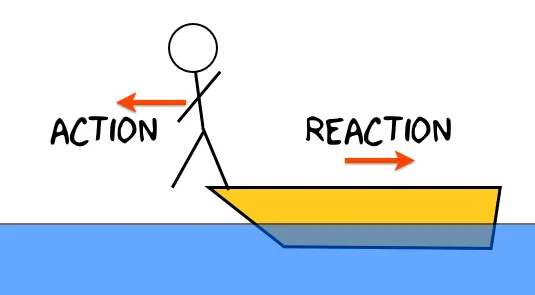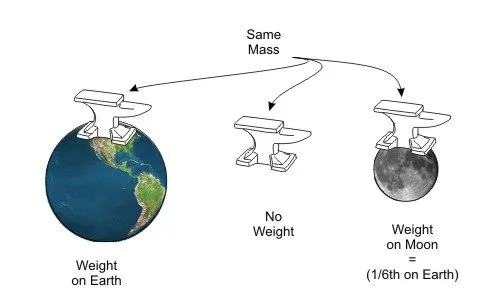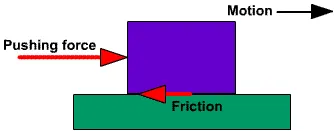# Physics - Classical mechanics - Newton's 3rd law and mass vs weightHello it's a me again drifter1! Today we continue with Physics by getting into Newton's 3rd law and also making the difference of mass and weight more clear.

I suggest you to read my previous post if you haven't already, cause it also covers what mass is.

So, without further do, let's get started!

## Mass vs Weight

Let's first start by covering the difference of mass and weight.

Mass is the quantity of matter an object has and represents it's inertia (inertial mass).

We covered more about mass last time, but one thing that is really important too is that mass doesn't change! Else we are talking about another object! So, mass is always the same if we talk about the same object in any part of the universe!

So, what is Weight?

Weight is the tractive force that the Earth (or Moon, Sun etc.) applies to another object.

On Earth the acceleration of gravity is about 9.8 m/s^2.

Suppose we have an 1kg object.

By applying Newton's 2nd law we get:

F = ma => W = mg => W = 1 * 9.8 => W = 9.8N

So, the tractive force of the Earth to that object is 9.8 N, but the mass of the object stays 1Kg!

Many people keep using those terms wrong and alternately like they are the same.

You shouldn't!

What does this mean for us?Well, on the Moon, where the gravity is 1.62 m/s^2 the object from before would "weight" (have a weight of) 1.62N, but the mass would be 1Kg again! And, because the gravity changes even on different places of the Earth (but not much to be worth considering most of the times) we might want to use another way of calculating mass by not using Newton's laws! The best way is by using a prototype mass that we know the mass for sure and calculating the mass of any other object from there.

Lastly, I also want to point out the concept of gravitational mass! This type of mass, that is actually a force and so the weight of an object, is used when we want to study the gravitanional interactions between objects! The problem with this type of mass is that it changes even on small movements and even differentiates on points of the same object. But either way some applications use it and it is sometimes pretty useful.

## Newton's 3rd Law

Let's now get to the main topic of this post.

Newton's 3rd and final law of motion is maybe the most important one.

Forces always come in pairs

This is how you would describe the law in one small sentence!

So small and simple, but so useful!

By studying the interactions of objects Newton found out that if we apply a Force to an object that object will apply the same force back to us (opposite force).

For each action there is a reaction.

Some also like to explain the law that way.

So, when applying a Force Fa to an object that object will apply a Force Fb = -Fa to us.

By solving this equation in one side we get:

Fa + Fb = 0

So, the vectorial addition of two forces in action/reaction is always zero!

Something important to note is that those two forces are not applied to the same object! Each one applies an force to the other so that the sum of those forces is zero, but this doesn't mean that the force applied to each of the objects is also zero!

Example

You might now think, well if I throw a feather I apply a force to the feather and the feather applies a force back to me, because of action/reaction, but where is that force? I don't feel anything!

Of course you don't feel it! You have a much bigger mass (I repeat mass) which means that your intertia is much bigger then the one of the feather's. The feather has a pretty small mass and so we don't need a lot of force to give it a lot of acceleration (visible result). We on the other hand have a big mass and so need a much bigger force to get a visible acceleration.

FrictionSomething worth noting too is that there is a friction (force) between our feet and the ground. The friction eliminates forces up to a specific point, that the feather of course don't got to apply to us. The force applied needs to be bigger then a specific limit to cancel out the friction.

We will get into friction more specifically later on, when we start getting into examples, but just to know something from now too, I would like to note that friction is somehow related to the gravity and the force the ground pushes us up, because of action/reaction. There is a factor that is related to the surface type and defines how "smooth" the surface is and that factor is multiplied with the force the ground applies to us.

So friction is:

F = μN

where F is the friction, μ is the surface factor and N is the the force of the ground to us.

This means that:

• On a horizontal plane N = -W (simple action/reaction) and so the weight "equals" the force of the ground.
• On a vertical plane we of course don't have any force of the ground (N) and so friction (F)
• On a slope plane we will find the vector components of weight and calculate N that way.

### Image Sources:### Newton's Laws:

Force and Netwon's first law

Mass and Newton's second law

Newton's 3rd law and mass vs weight -> This post! :P

And this is actually it! I hope you enjoyed it!

Next time in Physics we will get into how and when we apply all the Laws we covered!

Bye!

H2
H3
H4
3 columns
2 columns
1 column